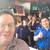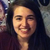0

# Totally Confused by L,T, R, B Coordinates for Random Animation Movement

Ted Ollikkala 6 years ago updated by Inna Komarovsky 6 years ago
Let's say I have a symmetrical object exactly centered on the screen at at 640, 400 (on an Android screen set for 1280 x 800) and I want to animate a random movement constrained ten pixels in each direction. What are the Left, Top, Right and Bottom coordinates that should be entered?,This is driving me crazy! :-)Hi Ted,

The constraints depend on the dimensions of the element as well as its coordinates. If the element's anchor point is "Middle-Center," then the constraints should be the parts of the screen where the element's edges should not pass.

I hope this helps : ) let me know if you'd like more information.Hi Ted,

The constraints depend on the dimensions of the element as well as its coordinates. If the element's anchor point is "Middle-Center," then the constraints should be the parts of the screen where the element's edges should not pass.

I hope this helps : ) let me know if you'd like more information.Thanks - it's a start, Inna :-) If the square in the center of the screen at (640, 400) were 100x100 and you wanted random movement 10 pixels in each direction, the element's anchor point could not be middle center, Is this correct? (No, wait...)L = 640 - 110
T = 400 - 100
R = 640 + 110
B = 400 + 100

The object is anchored in the center-middle
This assumes that the top left corner is (0,0), as the measurement icon indicates+1
Yes, the anchor point can be middle-center, and the top left corner of the screen is (0,0). If the dimensions of the element are 100x100, then actually to calculate the constraints you can use the following:
Left: 640 - 60 = 580
Top: 400 - 60 = 340
Right: 640 + 60 = 700
Bottom: 400 + 60 = 460
I use 60 because it is 10 plus half of the element's width (and height).I feel like such a math dummy (guess I'm about 20 years out of practice!) - thank you, Inna!You're welcome! : ) Glad to help